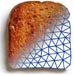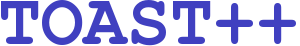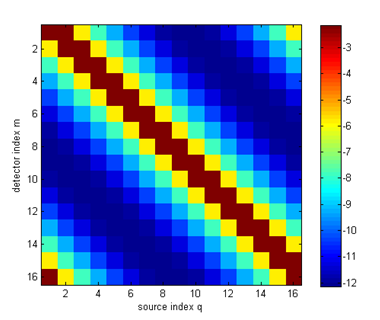Image Reconstruction in Diffuse Optical Tomography

# Tutorial list

Tutorial 1: Building a DOT forward solver
Tutorial 2: Building a DOT inverse solver

# Python module demos

The Toast-Python toolbox contains a series of interactive demos that allow to explore specific topics of the forward and inverse problems in diffuse optical tomography. The demos require a Python interpreter, as well as the NumPy, SciPy and matplotlib modules installed, in addition to the TOAST binary package and TOAST-Python bindings.

# Python online tutorials

Below is a list of online tutorials shows typical use examples for the Python-Toast toolbox. Each tutorial contains a link to the Python script to recreate the example.

The tutorials introduce the concept of the forward and inverse problems in optical tomography step by step. It is recommended to work through them in sequence, since later tutorials build upon the previous ones.## Tutorial 1: Building a DOT forward solver

This example shows a basic forward solver for a simple 2-D homogeneous problem, generating steady-state boundary data. It explains how to create a mesh, set up homogeneous parameter vectors, define the source and detector profiles, and solve the linear FEM problem.

## Tutorial 2: Building a DOT inverse solver

This example shows the reconstruction of absorption and scattering parameters from frequency-domain boundary data. It explains how to set up a nonlinear conjugate gradient solver with line search for minimising an objective function.